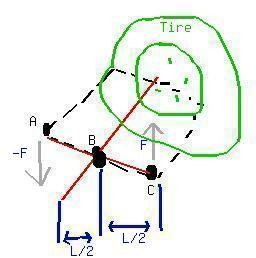Ask question

# Given: A parallelogram with diagonals equal to 56cm and 34cm. The intersect of the angles is 120^circ. Find the shorter side's length.# Given: A parallelogram with diagonals equal to 56cm and 34cm. The intersect of the angles is 120^circ. Find the shorter side's length.

Question
Non-right triangles and trigonometryasked 2021-03-08
Given:
A parallelogram with diagonals equal to 56cm and 34cm. The intersect of the angles is $$\displaystyle{120}^{\circ}$$. Find the shorter side's length.

## Answers (1)2021-03-09
Suggest the length of the shorter side is x. The $$\displaystyle{120}^{\circ}$$ angle is opposite to the longer side. So the angle opposite to the shorter side is $$\displaystyle{180}-{120}={60}^{\circ}$$. Now we have a triangle with one side being x, and the two others: 17cm and 28cm, because the diagonals of a parallelogram bisect each other. Use the Law of Cosines to solve this.
$$\displaystyle{x}^{{2}}={28}^{{2}}+{17}^{{2}}-{2}{\left({28}\right)}{\left({17}\right)}{{\cos{{60}}}^{\circ}}$$
$$\displaystyle{x}^{{2}}={597}$$
$$\displaystyle{x}=\sqrt{{597}}$$
$$\displaystyle{x}\approx{24.4}$$ cm
The shorter side is approximately 24.4cm long.

### Relevant Questionsasked 2020-11-24
A triangle is given with one side equal to 18m (a) and angles laying on this side equal to $$\displaystyle{48}^{\circ}$$ (A) and $$\displaystyle{37}^{\circ}$$ (B). Find the ramaining angle (C) and sides (b and c).asked 2021-02-03
Given a triangle with a $$\displaystyle{112}^{\circ}$$ angle. The adjusted sides to this angle are equal. Find their length, if $$\displaystyle{A}={80}{c}{m}^{{2}}$$.asked 2021-01-13
Given a triangle with all angles equal and sides equal to:
1. 3x-5
2. 4x-30
3. 2x+20
Find the value of x.asked 2021-02-11
Find the shorter diagonal of a parallelogram, if
l=18cm
w=26cm
$$\displaystyle\angle{a}={39}^{\circ}$$asked 2021-01-31
Given a triangles. One angle is $$\displaystyle{42}^{\circ}$$. Find the other angles if they're equal.asked 2020-11-26
Are triangles necessarily congruent, is:
1. Each side of one triangle is equal to one of the other triangle's sides.
2. If the two triangles are right and each cathetus of one triangle is equal to a cathetus from the other triangle.
3. If the angles of one triangle are equal to the angles of the other triangle.asked 2021-02-21
To prove: The similarity of $$\displaystyle\triangle{N}{W}{O}$$ with respect to $$\displaystyle\triangle{S}{W}{T}$$.
Given information: Here, we have given that NPRV is a parallelogram.asked 2021-03-26
A pair of forces with equal magnitudes, opposite directions,and different lines of action is called a "couple". When acouple acts on a rigid object, the couple produces a torque thatdoes not depend on the location of the axis. The drawing shows acouple acting on a tire wrench, each force being perpendicular tothe wrench. Determine an expression for the torque produced by thecouple when the axis is perpendicular to the tired and passesthrough (a) point A, (b) point B, and (c) point C. Express youranswers in terms of the magnitude F of the force and the length Lof the wrenchasked 2020-11-24
comprehension check for derivatives of trigonometric functions:
a) true or false: if $$\displaystyle{f}{p}{r}{i}{m}{e}{\left(\theta\right)}=-{\sin{{\left(\theta\right)}}},{t}{h}{e}{n}{f{{\left(\theta\right)}}}={\cos{{\left(\theta\right)}}}.$$
b) true or false: $$\displaystyle{I}{f}\theta$$ is one of the non right angles in a right triangle and ЗІЛsin(theta) = 2/3ZSK, then the hypotenuse of the triangle must have length 3.asked 2021-01-08
PQRS is a parallelogram. $$\displaystyle{m}∠{Q}={11}{x}+{13}$$ and $$\displaystyle{m}∠{S}={15}{x}-{23}$$. Find the measure of $$\displaystyle∠{P}$$.
...